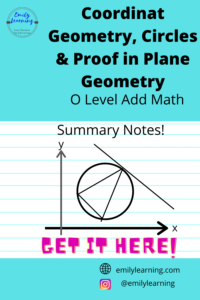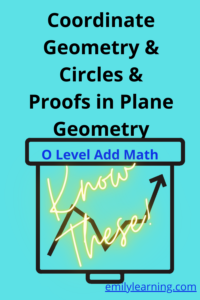# Tag: AMath Coordinate Geometry## Summary Notes for Coordinate Geometry, Circles and Proof in Plane Geometry – O Level Add Math

Here, you will find the summary notes for coordinate geometry, circles, and proof in plane geometry written based on O Level Additional Mathematics Syllabus. We…## What you need to know for coordinate geometry, circles and proofs in plane geometry tested in O Level Additional Mathematics

Let’s talk about what you need to know for Coordinate geometry, circles (in coordinate geometry) and proofs in plane geometry for O Level Additional Mathematics.…